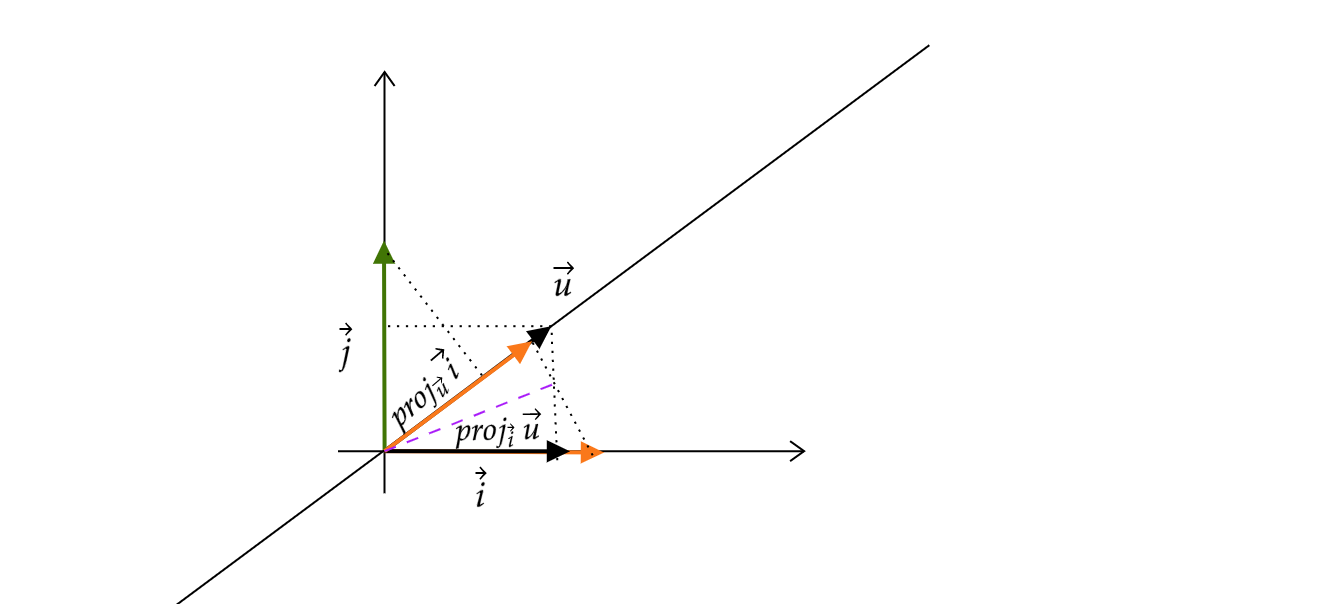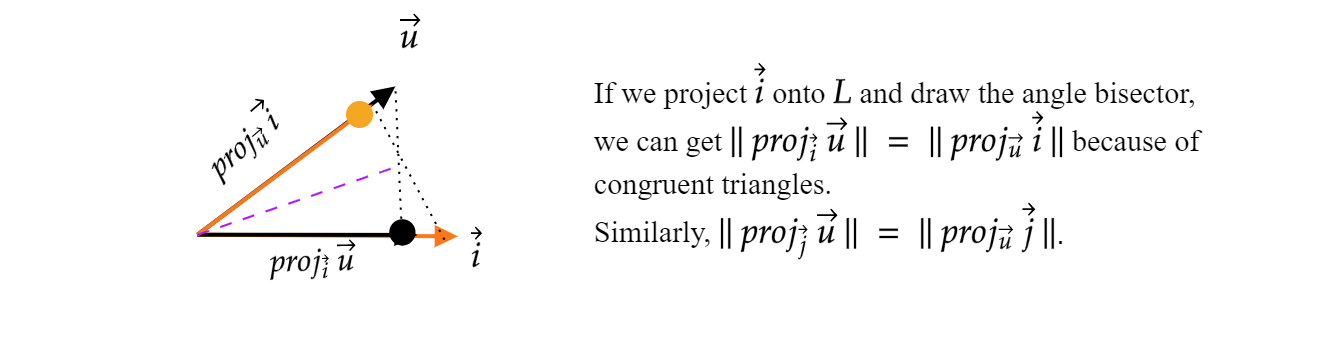# Sixian Li

## Dot products and projections

Linear Algebra

Last year, I watched 3Blue1Brown’s video of dot products, but I only had a vague idea about their connection to projections. However, dot products showed up again and again in my math courses, so I decided to rewatch it. To my relief, it makes much more sense after MATH223, and the connection is indeed “truly awesome” as 3B1B said in the video. Now, I’m going to reconstruct the whole idea to deepen my understanding. I created images with the help of Mathcha, an online math editor.

Multiplying a $1\times 2$ matrix $\begin{bmatrix} u_{x} & u_{y} \end{bmatrix}$ with a 2D($2\times 1$) vector $\begin{bmatrix} x\ y \end{bmatrix}$ gives us a $1\times 1$ scalar value $s\ =\ u_{x} x\ +u_{y} y.$We interpret this as “transforming any 2D vector to a point on the 1D number line”. Although this numerical value is just from the familiar matrix-vector multiplication, its geometric meaning is exciting. Questions What does multiplying by $\begin{bmatrix} u_{x} & u_{y} \end{bmatrix}$ mean geometrically? How is this related to taking the dot product with the vector $\begin{bmatrix} u_{x}\ u_{y} \end{bmatrix}$?Let $\vec{i} ,\ \vec{j}$ be unit vectors in $\mathbb{R}^{2}$, and let $\vec{u} \ =\begin{bmatrix} u_{x}\ u_{y} \end{bmatrix}$ be a unit vector on line $L$.But $| \ proj_{\vec{i}} \ \vec{u} \ |$ is just the x-coordinate of $\vec{u} ,\ u_{x}$. Now, if we want to know the orthogonal projection of any vector in $\mathbb{R}^{2}$ onto line $L$, we only need to find where $\vec{i} \ ,\ \vec{j}$ land. Note: The projection outputs a scalar, the orange dot on the number line, not a 2D vector lying on the line. What we get is the component of a vector in the direction $\vec{u}$. Define the transformation $T:\ \mathbb{R}^{2}\rightarrow \mathbb{R}$ as \begin{aligned}T(\begin{bmatrix}x \\ y\end{bmatrix}) =\begin{bmatrix}u_{x} & u_{y}\end{bmatrix}\begin{bmatrix}x\\y\end{bmatrix}\end{aligned}

which is the orthogonal projection onto line $L$. First, is it linear? Let $\vec{v} ,\ \vec{w} \ \in \mathbb{R}^{2} , c\ \in \mathbb{R} .$ \begin{aligned} T(\vec{0}) \ & =\ \begin{bmatrix} u_{x} & u_{y} \end{bmatrix}\begin{bmatrix} 0\\ 0 \end{bmatrix} \ =\ 0\\ & \\ T( c\vec{v} +\vec{w}) & =\ \begin{bmatrix} u_{x} & u_{y} \end{bmatrix}\begin{bmatrix} cv_{x} +w_{x}\\ cv_{y} +w_{y} \end{bmatrix}\\ & =\ u_{x}( cv_{x} +w_{x}) \ +\ u_{y}( cv_{y} +w_{y})\\ & =\ cv_{x} u_{x} \ +\ cv_{y} u_{y} +\ u_{x} w_{x} \ +u_{y} w_{y}\\ & \\ cT(\vec{v}) +T(\vec{w}) & =c\ \begin{bmatrix} u_{x} & u_{y} \end{bmatrix}\begin{bmatrix} v_{x}\\ v_{y} \end{bmatrix} \ +\ \begin{bmatrix} u_{x} & u_{y} \end{bmatrix}\begin{bmatrix} w_{x}\\ w_{y} \end{bmatrix}\\ & =\ c( u_{x} v_{x} +u_{y} v_{y}) \ +\ ( u_{x} w_{x} +u_{y} w_{y)} \end{aligned}

It is easy to see that (1) = (2), so it is linear. For any vector $\begin{bmatrix} x\\y \end{bmatrix} \in \mathbb{R}^{2} ,$ \begin{aligned}T\left(\begin{bmatrix}x\\y\end{bmatrix}\right) &=T\left( x\vec{i} \ +y\vec{j}\right) &=\ xT\left(\vec{i}\right) +yT\left(\vec{j}\right) &=\ xu_{x} \ +\ yu_{y}\end{aligned} which is the same as taking the dot product $\begin{bmatrix} x\ y \end{bmatrix} \cdot \begin{bmatrix} u_{x}\ u_{y} \end{bmatrix}$. What if $\vec{u}$ is not the unit vector? Then the unit vector will be $\vec{u}\frac{1}{||\ \vec{u} \ ||}$. So our matrix encoding the projection is now $\begin{bmatrix} \frac{u_{x}}{||\ \vec{u} \ ||} & \frac{u_{y}}{||\ \vec{u} \ ||} \end{bmatrix}$. \begin{aligned}T\left(\begin{bmatrix}x\\y\end{bmatrix}\right) \ & =\frac{xu_{x}}{||\ \vec{u} \ ||} \ +\ \frac{yu_{y}}{||\ \vec{u} \ ||}\\ ||\ \vec{u} \ ||\ T\left(\begin{bmatrix}x\\y\end{bmatrix}\right) \ & =xu_{x} \ +\ yu_{y}\end{aligned}\\ The left hand is multiplying the projection of $\begin{bmatrix} x\ y \end{bmatrix}$ onto $L$ by the length of $\vec{u}$, and the right hand side is $\begin{bmatrix} x\ y \end{bmatrix} \cdot \begin{bmatrix} u_{x}\ u_{y} \end{bmatrix}$

It is time to answer our questions:

• Geometrically, multiplying by $\begin{bmatrix} u_{x} & u_{y} \end{bmatrix}$ is the same as finding the component of any vector in direction $\vec{u} \ =\begin{bmatrix} u_{x}\ u_{y} \end{bmatrix}$ and scaling by $| \vec{u} |$. The lengthy version: projecting one vector onto the other one and multiplying by the length of the one being projected onto.
• Applying this transformation gives us the same result as taking the dot product with $\vec{u}$. This association is what he mentioned in the video as “duality”, a fascinating concept in math, so it’s definitely worth watching. Thanks again for the inspiration.